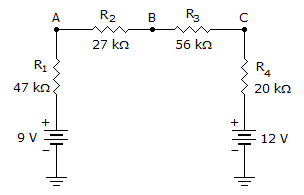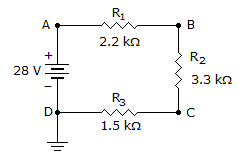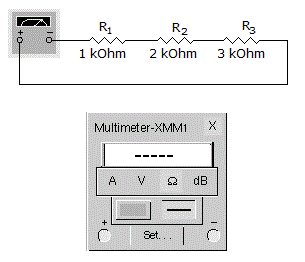# Electronics - Series Circuits

### Exercise :: Series Circuits - General Questions

26.Which is a true statement about the circuit in the given circuit?

 A. The batteries are series-aiding and the total source voltage equals 21 V. B. The batteries are series-opposing and the total source voltage equals 3 V. C. The batteries are series-aiding and the total source voltage equals 3 V. D. The batteries are series-opposing and the total source voltage equals 21 V.

Explanation:

No answer description available for this question. Let us discuss.

27.

A series circuit current:

 A. remains the same through each component B. is the same as the individual resistances C. is subtracted by each component D. varies through each component

Explanation:

No answer description available for this question. Let us discuss.

28.If R2 opens in the given circuit, what is the voltage at point B?

 A. +19.2 V B. +8.8 V C. +28 V D. –19.2 V

Explanation:

No answer description available for this question. Let us discuss.

29.

If 5 V and 16 V power supplies are connected in series-opposing, what is the total voltage?

 A. 11 V B. 16 V C. 21 V D. 80 V

Explanation:

No answer description available for this question. Let us discuss.

30.

What is the total resistance?A. 0 k; there is no current B. 2 k; the average C. 3 k; the largest D. 6 k; the sum